Floyd-Warshall Algorithm

# Floyd-Warshall Algorithm

#### In this tutorial, you will learn how floyd-warshall algorithm works. Also, you will find working examples of floyd-warshall algorithm in C, C++, Java and Python.

Floyd-Warshall Algorithm is an algorithm for finding the shortest path between all the pairs of vertices in a weighted graph. This algorithm works for both the directed and undirected weighted graphs. But, it does not work for the graphs with negative cycles (where the sum of the edges in a cycle is negative).

A weighted graph is a graph in which each edge has a numerical value associated with it.

Floyd-Warhshall algorithm is also called as Floyd's algorithm, Roy-Floyd algorithm, Roy-Warshall algorithm or WFI algorithm.

This algorithm follows the dynamic programming approach to find the shortest paths.

## How Floyd-Warshall Algorithm Works?

Let the given graph be: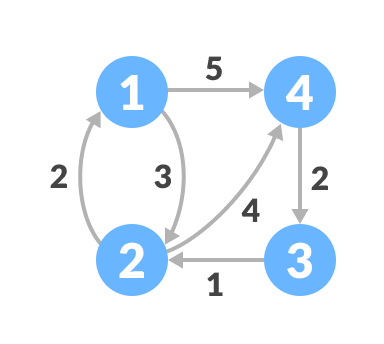Follow the steps below to find the shortest path between all the pairs of vertices.

1. Create a matrix `A1` of dimension `n*n` where n is the number of vertices. The row and the column are indexed as i and j respectively. i and j are the vertices of the graph.

Each cell A[i][j] is filled with the distance from the `ith` vertex to the `jth` vertex. If there is no path from `ith` vertex to `jth` vertex, the cell is left as infinity.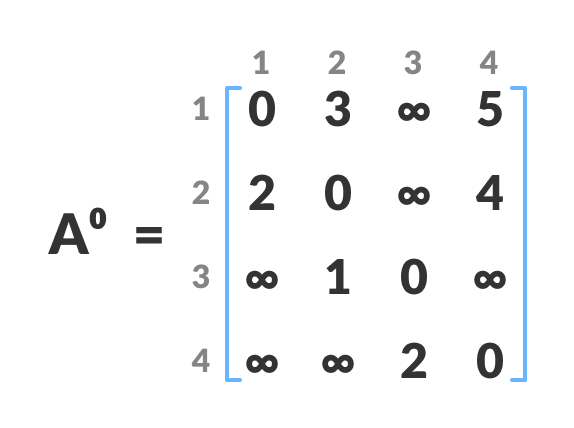2. Now, create a matrix `A1` using matrix `A0`. The elements in the first column and the first row are left as they are. The remaining cells are filled in the following way.

Let k be the intermediate vertex in the shortest path from source to destination. In this step, k is the first vertex.` A[i][j]` is filled with `(A[i][k] + A[k][j]) if (A[i][j] > A[i][k] + A[k][j])`.

That is, if the direct distance from the source to the destination is greater than the path through the vertex k, then the cell is filled with `A[i][k] + A[k][j]`.

In this step, k is vertex 1. We cacluate the distance from source vertex to destination vertex through this vertex k.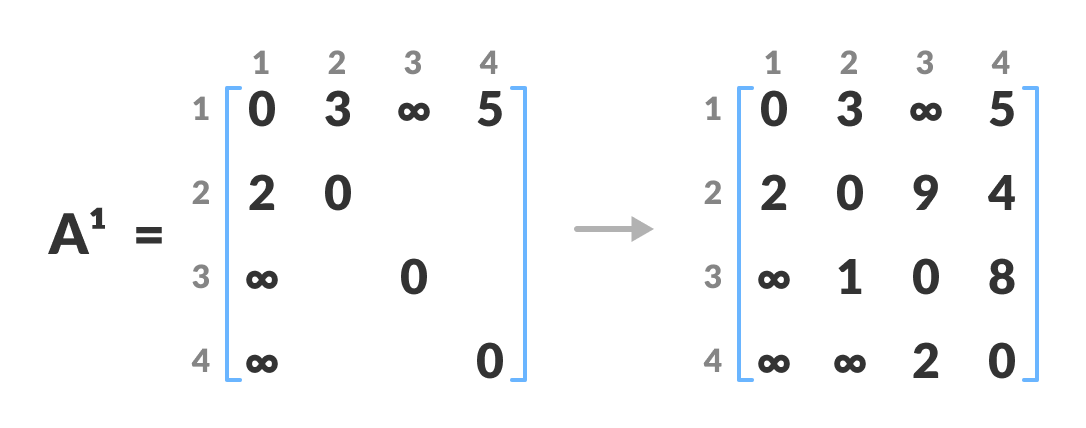For example: For `A1[2, 4]`, the direct distance from vertex 2 to 4 is 4 and the sum of the distance from vertex 2 to 4 through vertex (ie. from vertex 2 to 1 and from vertex 1 to 4) is 7. Since `4 < 7`, `A0[2, 4]` is filled with 4.
3. In a similar way, `A2` is created using `A3`. The elements in the second column and the second row are left as they are.

In this step, k is the second vertex (i.e. vertex 2). The remaining steps are the same as in step 2.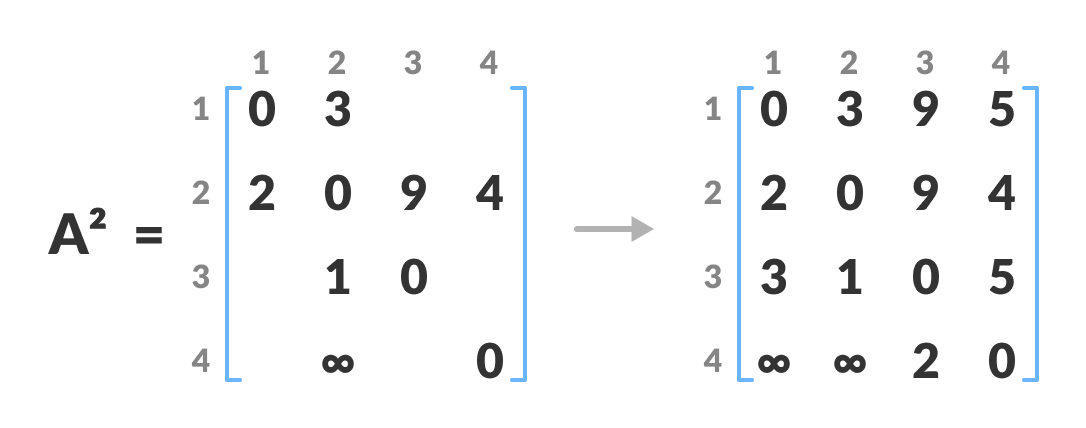4. Similarly, `A3` and `A4` is also created.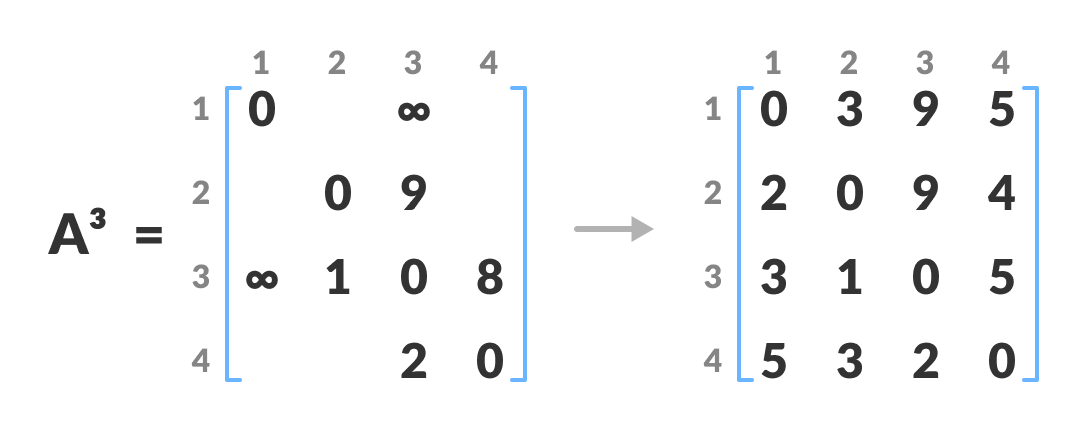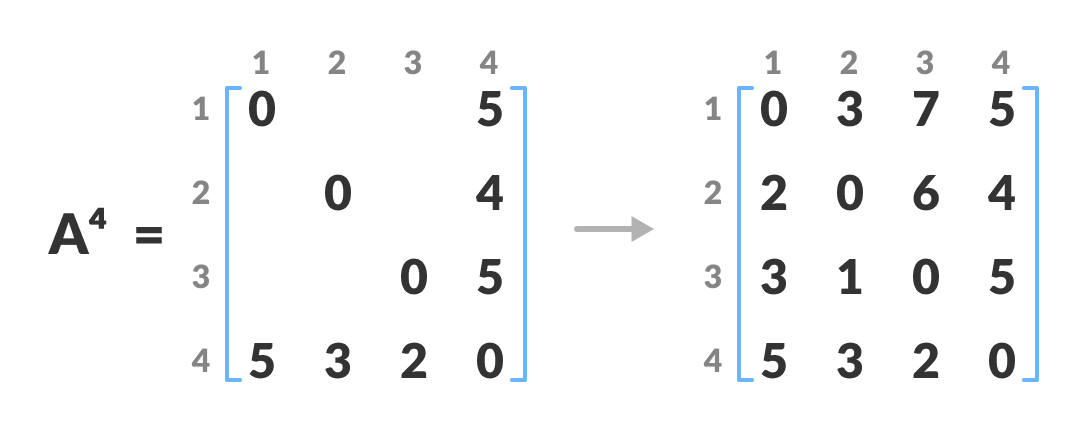5. `A4` gives the shortest path between each pair of vertices.

## Floyd-Warshall Algorithm

```n = no of vertices
A = matrix of dimension n*n
for k = 1 to n
for i = 1 to n
for j = 1 to n
Ak[i, j] = min (Ak-1[i, j], Ak-1[i, k] + Ak-1[k, j])
return A
```

## Python, Java and C/C++ Examples

``````# Floyd Warshall Algorithm in python

# defining the number of vertices
nV = 4

INF = 999

def floydWarshall(graph):
dist = list(map(lambda i : list(map(lambda j : j, i)), graph) )

for k in range(nV):
for i in range(nV):
for j in range(nV):
dist[i][j] = min(dist[i][j], dist[i][k]+ dist[k][j])
printSolution(dist)

def printSolution(dist):
for i in range(nV):
for j in range(nV):
if(dist[i][j] == INF):
print("INF", end =" ")
else:
print(dist[i][j], end ="  ")
print(" ")

graph = [[0, 3, INF, 5],
[2, 0, INF, 4],
[INF, 1, 0, INF],
[INF, INF, 2, 0]];
floydWarshall(graph);``````
``````// Floyd Warshall Algorithm in Java

import java.util.*;
import java.lang.*;
import java.io.*;

class FloydWarshall {
final static int INF = 999, nV = 4;

void floydWarshall(int graph[][]) {
int A[][] = new int[nV][nV];
int i, j, k;

for (i = 0; i < nV; i++)
for (j = 0; j < nV; j++)
A[i][j] = graph[i][j];

for (k = 0; k < nV; k++) {
for (i = 0; i < nV; i++) {
for (j = 0; j < nV; j++) {
if (A[i][k] + A[k][j] < A[i][j])
A[i][j] = A[i][k] + A[k][j];
}
}
}
printMatrix(A);
}

void printMatrix(int A[][]) {
for (int i = 0; i < nV; ++i) {
for (int j = 0; j < nV; ++j) {
if (A[i][j] == INF)
System.out.print("INF ");
else
System.out.print(A[i][j] + "  ");
}
System.out.println();
}
}

public static void main(String[] args) {
int graph[][] = { { 0, 3, INF, 5 },
{ 2, 0, INF, 4 },
{ INF, 1, 0, INF },
{ INF, INF, 2, 0 } };
FloydWarshall a = new FloydWarshall();
a.floydWarshall(graph);
}
}``````
``````// Floyd-Warshall Algorithm in C

#include <stdio.h>

// defining the number of vertices
#define nV 4

#define INF 999

void printMatrix(int A[][nV]);

void floydWarshall(int graph[][nV])
{
int A[nV][nV], i, j, k;

for (i = 0; i < nV; i++)
for (j = 0; j < nV; j++)
A[i][j] = graph[i][j];

for (k = 0; k < nV; k++)
{
for (i = 0; i < nV; i++)
{
for (j = 0; j < nV; j++)
{
if (A[i][k] + A[k][j] < A[i][j])
A[i][j] = A[i][k] + A[k][j];
}
}
}
printMatrix(A);
}

void printMatrix(int A[][nV])
{
for (int i = 0; i < nV; i++)
{
for (int j = 0; j < nV; j++)
{
if (A[i][j] == INF)
printf("%4s", "INF");
else
printf("%4d", A[i][j]);
}
printf("\n");
}
}

int main()
{
int graph[nV][nV] = {{0, 3, INF, 5},
{2, 0, INF, 4},
{INF, 1, 0, INF},
{INF, INF, 2, 0}};
floydWarshall(graph);
}``````
``````// Floyd Warshall Algorithm in C++

#include <iostream>
using namespace std;

// defining the number of vertices
#define nV 4

#define INF 99999

void printMatrix(int A[][nV]);

void floydWarshall(int graph[][nV])
{
int A[nV][nV], i, j, k;

for (i = 0; i < nV; i++)
for (j = 0; j < nV; j++)
A[i][j] = graph[i][j];

for (k = 0; k < nV; k++)
{
for (i = 0; i < nV; i++)
{
for (j = 0; j < nV; j++)
{
if (A[i][k] + A[k][j] < A[i][j])
A[i][j] = A[i][k] + A[k][j];
}
}
}
printMatrix(A);
}

void printMatrix(int A[][nV])
{
for (int i = 0; i < nV; i++)
{
for (int j = 0; j < nV; j++)
{
if (A[i][j] == INF)
cout << "INF"
<< "  ";
else
cout << A[i][j] << "  ";
}
cout << endl;
}
}

int main()
{
int graph[nV][nV] = {{0, 3, INF, 5},
{2, 0, INF, 4},
{INF, 1, 0, INF},
{INF, INF, 2, 0}};
floydWarshall(graph);
}``````

## Floyd Warshall Algorithm Complexity

### Time Complexity

There are three loops. Each loop has constant complexities. So, the time complexity of the Floyd-Warshall algorithm is `O(n3)`.

### Space Complexity

The space complexity of the Floyd-Warshall algorithm is `O(n2)`.

## Floyd Warshall Algorithm Applications

• To find the shortest path is a directed graph
• To find the transitive closure of directed graphs
• To find the Inversion of real matrices
• For testing whether an undirected graph is bipartite